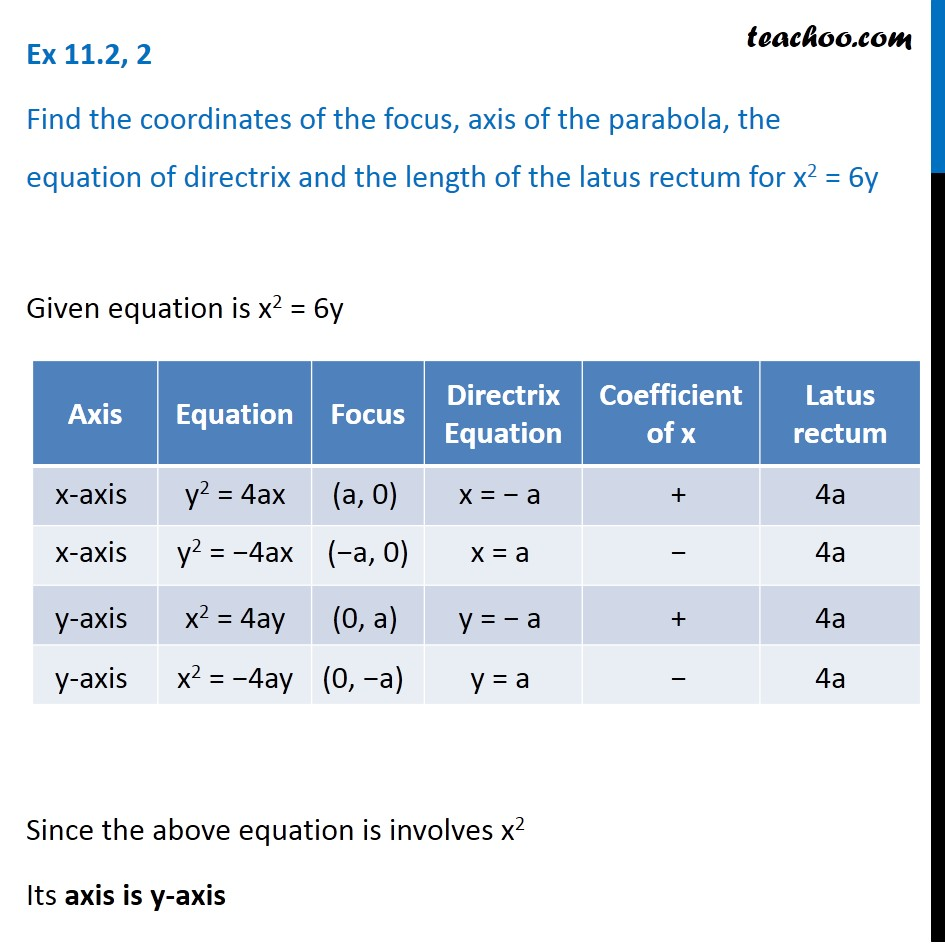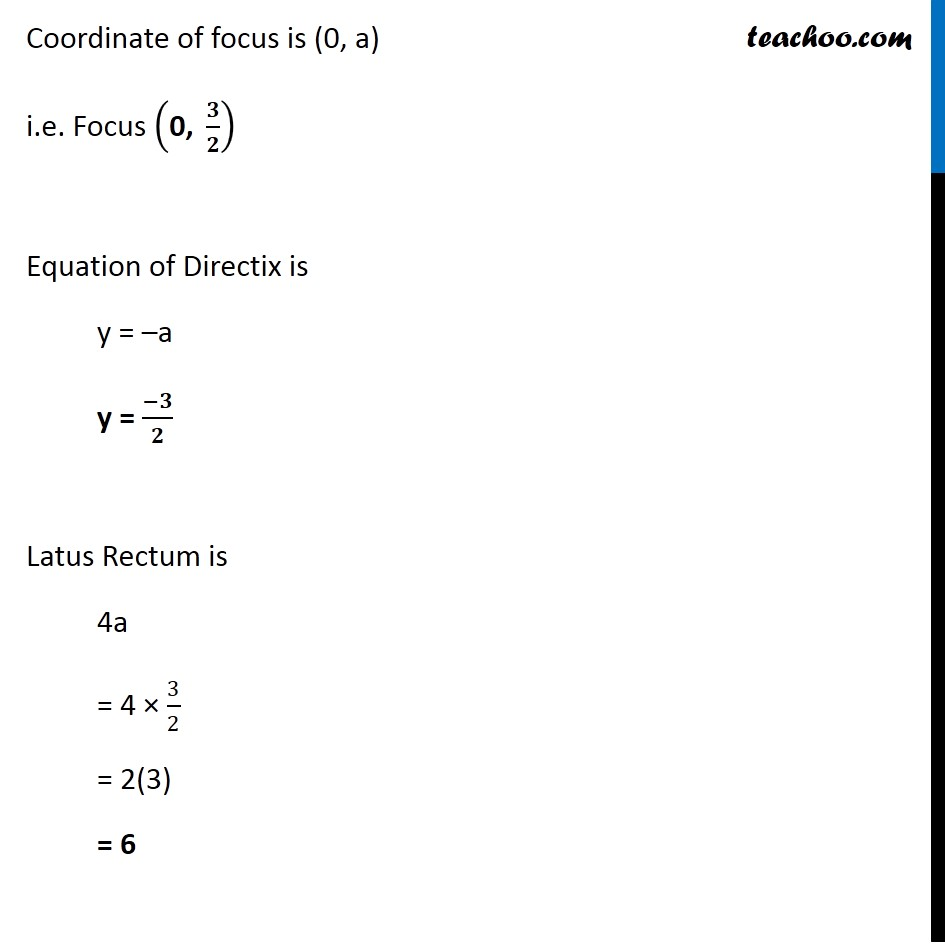Ex 10.2

Chapter 10 Class 11 Conic Sections
Serial order wiseLearn in your speed, with individual attention - Teachoo Maths 1-on-1 Class

### Transcript

Ex 10.2, 2 Find the coordinates of the focus, axis of the parabola, the equation of directrix and the length of the latus rectum for x2 = 6y Given equation is x2 = 6y Since the above equation is involves x2 Its axis is y-axis Also coefficient of x is positive (+1) Hence we use equation x2 = 4ay Comparing x2 = 6y & x2 = 4ay Comparing (1) & (2) 4ay = 6y a = 6𝑦/4𝑦 a = 3/2 Coordinate of focus is (0, a) i.e. Focus ("0, " 𝟑/𝟐) Equation of Directix is y = –a y = (−𝟑)/𝟐 Latus Rectum is 4a = 4 × 3/2 = 2(3) = 6